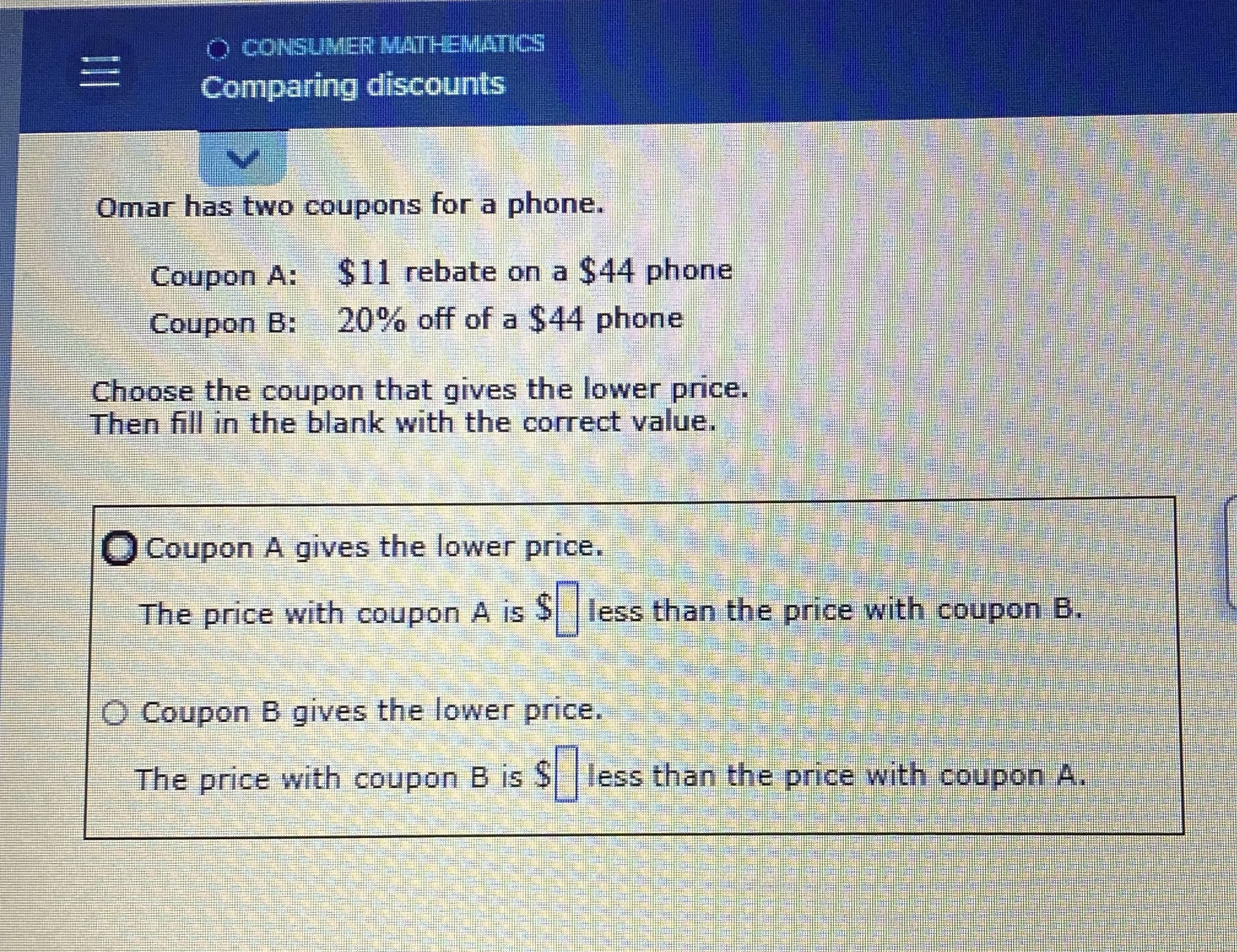### Still have math questions?

Algebra
QuestionOmar has two coupons for a phone.

Coupon A: $$\ 11$$ rebate on a $$\ 44$$ phone

Coupon B: $$20 \%$$ off of a $$\ 44$$ phone

Choose the coupon that gives the lower price.

Then fill in the blank with the correct value.

Coupon A gives the lower price.

The price with coupon $$A$$ is $$\ \square$$ less than the price with coupon B.

Coupon B gives the lower price.

The price with coupon $$B$$ is $$\ \square$$ less than the price with coupon A.